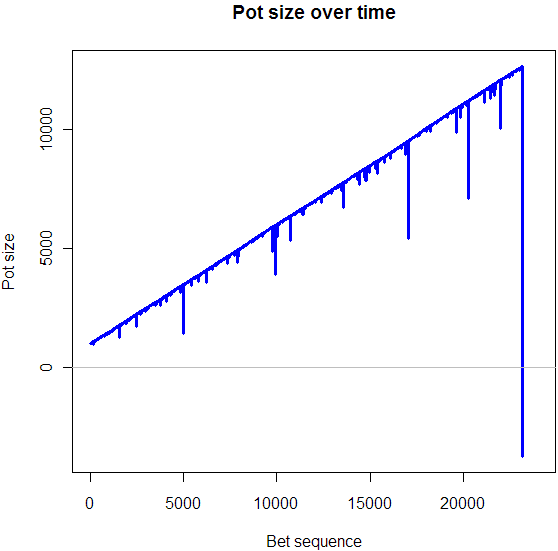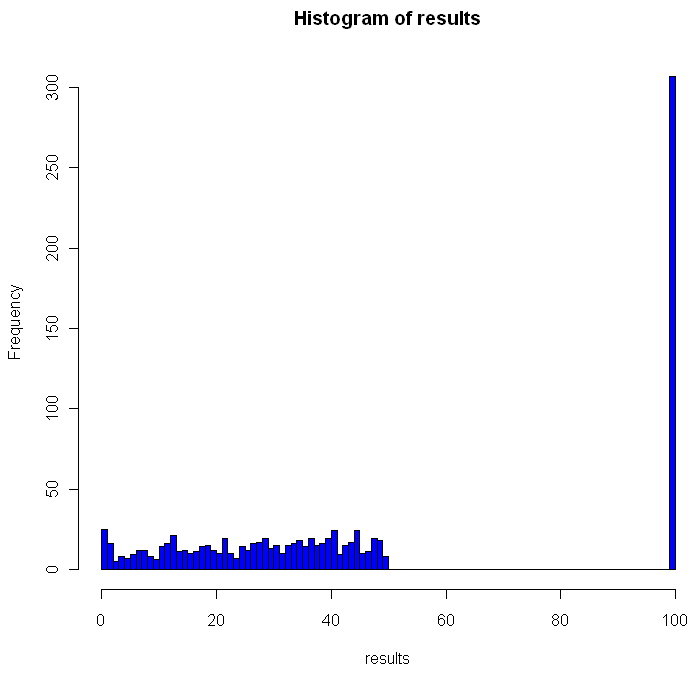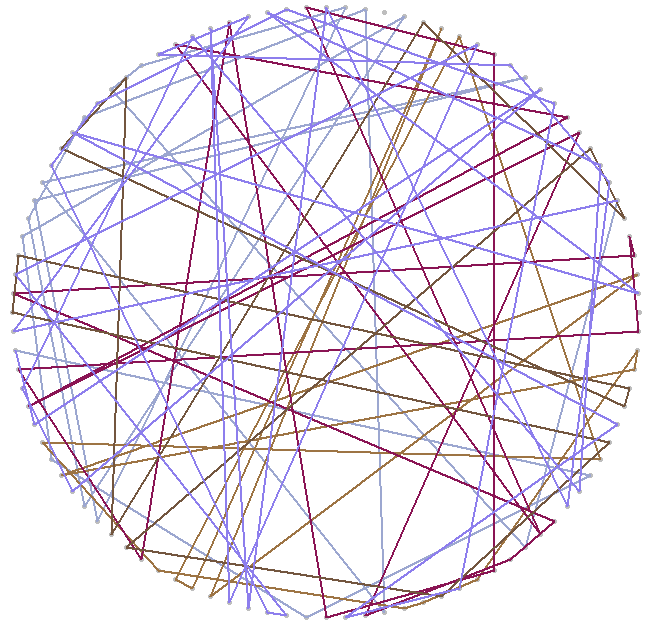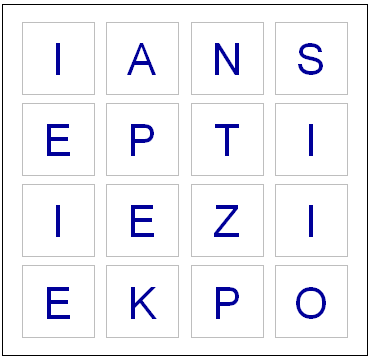1
Dec 11

## Wasting away again in MartingalevilleAlright, I better start with an apology for the title of this post. I know, it’s really bad. But let’s get on to the good stuff, or, perhaps more accurately, the really frightening stuff. The plot shown at the top of this post is a simulation of the martingale betting strategy. You’ll find code for it here. What is the martingale betting strategy? Imagine you go into a a mythical casino that gives you perfectly fair odds on the toss of a mythically perfect coin. You can bet one dollar or a million. Heads you lose the amount you bet, tails you win that same amount. For your first bet, you wager $1. If you win, great! Bet again with a dollar. If you lose, double your wager to$2. Then if you win the next time, you’ve still won $1 overall (lost$1 then won $2). In general, continue to double your bet size until you get a win, then drop your bet size back down to a dollar. Because the probably of an infinite loosing streak is infinitely small, sooner or later you’ll make$1 off of the sequence of bets. Sound good?

The catch (you knew there had to be a catch, right?) is that the longer you use the martingale strategy, the more likely you are to go broke, unless you have an infinitely large bankroll. Sooner or later, a run of heads will wipe out your entire fortune. That’s what the plot at the beginning of this post shows. Our simulated gambler starts out with $1000, grows her pot up to over$12,000 (with a few bumps along the way), then goes bankrupt during a single sequence of bad luck. In short, the martingale stagy worked spectacularly well for her (12-fold pot increase!) right up until the point where it went spectacularly wrong (bankruptcy!).

Pretty scary, no? But I haven’t even gotten to the really scary part. In an interview with financial analyst Karl Denninger, Max Keiser explains the martingale betting strategy then comments:

“This seems to be what every Wall Street firm is doing. They are continuously loosing, but they are doubling down on every subsequent throw, because they know that they’ve got unlimited cash at their disposal from The Fed… Is this a correct way to describe what’s going on?

Karl Denninger replies. “I think it probably is. I’m familiar with that strategy. It bankrupts everyone who tries it, eventually…. and that’s the problem. Everyone says that this is an infinite sum of funds from the Federal Reserve, but in fact there is no such thing as an infinite amount of anything.”

Look at the plot at the beginning of this post again. Imagine the top banking executives in your country were paid huge bonuses based on their firm’s profits, and in the case of poor performance they got to walk away with a generous severance package. Now imagine that these companies could borrow unlimited funds at 0% interest, and if things really blew up they expected the taxpayers to cover the tab through bailouts or inflation. Do you think this might be a recipe for disaster?

9
Jul 10

## 100 Prisoners, 100 lines of codeIn math and economics, there is a long, proud history of placing imaginary prisoners into nasty, complicated scenarios. We have, of course, the classic Prisoner’s Dilemma, as well as 100 prisoners and a light bulb. Add to that list the focus of this post, 100 prisoners and 100 boxes.

In this game, the warden places 100 numbers in 100 boxes, at random with equal probability that any number will be in any box. Each convict is assigned a number. One by one they enter the room with the boxes, and try to find their corresponding number. They can open up to 50 different boxes. Once they either find their number or fail, they move on to a different room and all of the boxes are returned to exactly how they were before the prisoner entered the room.

The prisoners can communicate with each other before the game begins, but as soon as it starts they have no way to signal to each other. The warden is requiring that all 100 prisoners find their numbers, otherwise she will force them to listen to hundreds of hours of non-stop, loud rock musician interviews. Can they avoid this fate?

The first thing you might notice is that if every prisoner opens 50 boxes at random, they will have a $0.5$ probability of finding their number. The chances that all of them will find their number is $(\frac{1}2)^{100}$, which is approximately as rare as finding a friendly alien with small eyes. Can they do better?

Of course they can. Otherwise I wouldn’t be asking the question, right? Before I explain how, and go into a Monte Carlo simulation in R, you might want to think about how they can do it. No Googling!

All set? Did you find a better way? The trick should be clear from the code below, but if not skip on to the explanation.

# How many times should we run this experiment?
iters = 1000
results = rep(0,iters)

for(i in 1:iters) {
# A random permutation:
boxes = sample(1:100,100)

# Labels for our prisoners
prisoners = 1:100

# Track how many "winners" we have
foundIt = 0

# Main loop over the prisoners
for(prisoner in prisoners) {

# Track the prisoners path
path = c(prisoner)

tries = 1

# Look first in the box that matches your own number
inBox = boxes[prisoner]

while(tries < 50) { 			 			path = c(path, inBox) 			 			if(inBox == prisoner) { 				#cat("Prisoner", prisoner, "found her number on try", tries, "\n") 				foundIt = foundIt + 1 				break; 			} else { 				# Follow that number to the next box 				inBox = boxes[inBox] 			} 			tries = tries+1 		} 		 		# cat("Prisoner", prisoner, "took path", paste(path, collapse=" -> "), "\n")
}

# How many prisoners found their numbers?
cat("A total of", foundIt, "prisoners found their numbers.\n")
flush.console()
results[i] = foundIt
}

hist(results, breaks=100, col="blue")


Here is what one of my plots looked like after running the code:Out of the 1000 times I ran the experiment, on 307 occasions every single prisoner found his number. The theoretical success rate is about 31%. So, if it’s not clear from the code, what was the strategy employed by the prisoners and how does it work?

One way to look at the distribution of numbers in boxes is to see it as a permutation of the numbers from 1 to 100. Each permutation can be partitioned into what are called cycles. A cycle works like this: pick any number in your permutation. Let’s say it’s 23. Then you look at the number the 23rd place (ie the number in the 23rd box, counting from the left). If that number is 16, you look at the number in the 16th place. If that number is 87, go open box number 87 and follow that number. Eventually, the box you open up will have the number that brings you back to where you started, completing the cycle. Different permutations have different cycles.

The key for the prisoner is that by starting with the box that is the same place from the left as his number, and by following the numbers in the boxes, the prisoner guarantees that if he is in a cycle of length less than 50, he will eventually open the box with his number in it, which would complete the cycle he began. One way to envision cycles of different lengths is to think about the extreme cases. If a particular permutation shifted every single number over one to the left (and wrapped number 1 onto the end), you would have a single cycle of length 100. Box 1 would contain number 2, box 2 number 3 and so on. On the other hand, if a permutation flipped every pair of consecutive numbers, you would have 50 cycles, each of length 2: box 1 would have number 2, box 2 would have number 1. Of course if your permutation doesn’t change anything you have 100 cycles of length 1.

As you can see from the histogram, when using this strategy you can never have between 50 and 100 winning prisoners. Anytime you have a single cycle of length greater than 50, for example 55, then all 55 prisoners who start on that cycle will fail to find their number. If no cycles are longer than 50, everyone wins. Just how rare are different cycles of different lengths? For the math behind that check out this excellent explanation by Peter Taylor of Queen’s University.

Before moving on I wanted to visualize these cycles. Try running the code below:

# Unit circle
plot(0,0,xlim=c(-1,1),ylim=c(-1,1),col="white",ann=FALSE, xaxt="n", yaxt="n")
for(i in 1:100) {
points(cos(2*i/100*pi), sin(2*i/100*pi),pch=20,col="gray")
}

mySample = sample(1:100,100)
for(i in 1:100) {
found = FALSE
nextItem = i

# Pick a random color for this cycle
color = sample(c(0:9,"A","B","C","D","E","F"),12,replace=T)
lineColor = paste("#", paste(color[1:6],collapse=""),sep="")

while(!found) {
# Draw the cycle

segments(cos(nextItem/50*pi), sin(nextItem/50*pi), cos(mySample[nextItem]/50*pi), sin(mySample[nextItem]/50*pi),col=lineColor,lwd=2)
Sys.sleep(.4)
if(mySample[nextItem] == i) {
found = TRUE
} else {
nextItem = mySample[nextItem]
}
}
}


You can adjust the “Sys.sleep()” parameter to make the animation faster. I recommend running the code to see how the cycles “develop” over time, but here’s a snapshot of what I got:2
Jul 10

## Word games in probability and RLast night, while playing Boggle, we ended up with a board without a single vowel. Not even a “Y” or “Qu”. This seemed fairly unusual, so I wondered what the chances were of such an occurrence. I found an online list of the letters each die has, and I could have written down the number of vowels on each one by hand, but whenever possible I like to do things by computer. So I fired up Hal and asked for some help with the calculations.

Apparently some European boards use a 5 x 5 grid, but here in the land of the Maple leaf our board has 16 cubes. Here are the letters on them, as I coded them into R:

d1 = c('S','R','E','L','A','C')
d2 = c('D','P','A','C','E','M')
d3 = c('Qu','B','A','O','J','M')
d4 = c('D','U','T','O','K','N')
d5 = c('O','M','H ','R','S','A')
d6 = c('E','I','F','E','H','Y')
d7 = c('B','R','I','F','O','X')
d8 = c('R','L','U','W','I','G')
d9 = c('N','S','O','W','E','D')
d10 = c('Y ','L','I','B','A','T')
d11 = c('T','N','I','G','E','V')
d12 = c('T','A','C','I','T','O')
d13 = c('P','S','U','T','E','L')
d14 = c('E','P','I','S','H ','N')
d15 = c('Y','K','U','L','E','G')
d16 = c('N','Z','E','V','A','D')


So now I had to check how many vowels were on each die. Here’s the code I used for this:

vowels = c('A','E','I','O','U','Qu','y')
vowelsFound = rep(0,16)
for(i in 1:16) {
found = 0
die = eval(parse(text=paste("d",i,collapse="",sep="")))
for(l in die) {
# Check to see if this letter is in the vowels vector
if(l %in% vowels) {
found = found + 1
}
}
vowelsFound[i] = found
}

# Probabilities of getting a vowel for each die
pVowels = vowelsFound/6

# Probability of getting no vowel for each die
pNoVowels = 1 - pVowels

# Chance that we will get not a single vowel, including "y" and "Qu"
print(prod(pNoVowels))


If you run the code above, you should see that the probability of getting no vowels (including “Y” and “Qu”) is 0.000642. That works out to one in every 1557 boards. So it’s quite rare, but by no means so extraordinary that it crosses the Universal probability bound. Also, it’s not enough to just calculate how rare your event is, or how rare any similar or more extreme event is, and then be astounded. You also have to include all the other possible events that would have left you amazed. What about getting all vowels (much more rare)? What about getting 8 or 9 E’s, or a row or column of all A’s or E’s? It’s likely that if you add up all probabilities of all the rare events which might leave you amazed, you’ll end up with a good chance of amazement every time.

I could have stopped here, but having coded the dice, I decided to create a simplified version of the game in R. If I have a chance over the next few days I’ll add some more features.

# You will need to download a dictionary file. I found one here:
# http://svn.pietdepsi.com/repos/projects/zyzzyva/trunk/data/words/north-american/owl2-lwl.txt
words = read.table("wordlistData.dat", colClasses = "character")
words = unlist(words[,1])

# Create a random board. Plot it.
board = diag(4)
dice = sample(1:16,16)
cntr = 4
for(i in dice) {
die = eval(parse(text=paste("d",i,collapse="",sep="")))
board[floor(cntr/4), (cntr %% 4) + 1] = sample(die,1)
cntr = cntr + 1
}

plot(0,0,xlim=c(0,4),ylim=c(0,4),col="white",ann=FALSE, xaxt="n", yaxt="n" )

for(m in 1:4) {
for(n in 1:4) {
text(m-.5,n-.5,labels=board[m,n],cex=2.75,col="#000099")
# Draw a square the easy way
points(m-.5,n-.5,pch=0,cex=10,lwd=1.5,col="gray")
}
}

# How many seconds to give for each round
gameTime = 180

START_TIME = proc.time()
elapsed = 0

# Simple scoring, with 1 point per letter.
# Dictionary only has words length 3 or longer
score = 0

cat("Find words. Hit enter after each word.\n")
while(elapsed < gameTime) {
myWord = scan(n=1, what=character()) # Get a single word
elapsed = signif(proc.time() - START_TIME, digits=4)
if (length(myWord)==0) {
cat("You have", gameTime - elapsed, "seconds left. Keep going!\n")
} else {

if(elapsed < gameTime) {
# Check if it's a real word, see if it is in dictionary
# Convert their guess to uppercase letter
myWord = toupper(myWord)

# If it is in the dictionary, give them points
if(myWord %in% words) {
# Make sure they haven't used this word before TODO

# Add it to their score
score = score + nchar(myWord)
cat("Congratulations. You are up to", score, "points.")
cat("You have", gameTime - elapsed, "seconds left. Keep going!\n")
} else {
# If it isn't in the dictionary, let the user know that they got it wrong.
cat("Sorry, that is not in the dictionary. Keep trying!\n")
}

}
}
}

cat("Out of time! ")
cat("Your final score was:", score, "points.")


Enjoy the game. Let me know if you notice any issues or have suggestions!

2
Jun 10

## It’s your move

I’m thinking of two numbers between 0 and 1. Your goal is to guess a number which falls in between my two numbers. Each guess costs you $1, and if you guess correctly you win the reciprocal of the length of my range (ie if I am thinking of 0.2 and 0.4, a correct guess wins you$5). At any time you may request that I choose of a new pair of numbers, and of course I will pick a new pair of numbers whenever you win.

What’s your strategy? Under certain conditions, this game is fair. How might you be able to have a positive expectation?

28
May 10

## The guessing game in R (with a twist, of course)

Maybe you remember playing this one as a kid. If you are about my age, you may have even created a version of this game as one of your first computer programs. You guess a number, the computer tells you if you if you are too low or high. I’ve limited the number of maximum guesses, and randomized the computer’s choice based on the Poisson distribution (more on that later).

Here’s the code. This was part of my attempt to understand how R reads input from the command line. One of the things I learned: you may need to save this to a file and run it with “source()”, instead of running it directly from the console, line by line.

# Classic guessing game with twist
x = 0
gotRight = 0
failed = 0

# Initial lambda for our random var
correct = 2000
initial = correct

# How many guesses should we allow per number
maxGuesses = 7

while(x != Inf) {
# The +1 part makes sure we never get zero, which would trigger 0's forever
correct = rpois(1,correct) + 1

# The advantage of using "cat" instead of "print" is that you remove those pesky quotation marks
cat("I am thinking of a number between 1 and infinity. What is it? (Type Inf to quit)\n")

# Solicit input from the user
x = scan(n=1) # Just one item in this vector

# Be nice and let the user quit.
if(x == Inf) {
cat("The correct answer was", correct, "\n")
cat("You got", gotRight, "right and failed", failed, "times. Maximum allowed guesses was", maxGuesses, "and initial lambda was", initial, ". Goodbye.\n")
cat("Post your score to http://www.statisticsblog.com/2010/05/the-guessing-game-in-r-with-a-twist-of-course/#comments \n")
break
}

for(i in 1:maxGuesses) {
if(x == correct) {
print("You rock!")
gotRight = gotRight + 1
break
} else {
if(i == maxGuesses) {
cat("You ran out of guesses. I will pick a new random number based on the last one.\n")
failed = failed + 1
} else {
if(x < correct) {
cat("You are too low. Guess again.\n")
} else {
cat("You are too high. Guess again.\n")
}

x = scan(n=1)
}
}
}
}


Note 1: My code makes a couple uses of the aparently controversial "break" function. I can still recall a heated debate I had with a CS professor who believed that calling "break" (in Python) was as bad as crossing the streams of your Proton Pack. That said, I have sucessfully used it on several occasions now without any appearance by Stay Puft Marshmallow Man or changing the natural order between dogs and cats. In R, the biggest problem with using constructs like "break" and "while" is that, for reasons clear only to readers of this blog but not myself, if you ask R for help about either of these tokens using

?term

you get an sent an error or to purgatory, respectively.

Hint: Because the random guesses are Poisson based, using a "half the distance" strategy for guessing may not be the best way to go. The hardcore amongst yourselves might want to calculate the median of the expected value conditional on having guessed too low or high.

Note 2: The Poisson isn't a very good distribution for for this. Maybe you can find a better one, or at least jack up the dispersion like an overzealous offroader tweaking the suspension of his 4Runner.